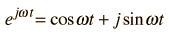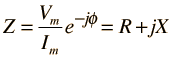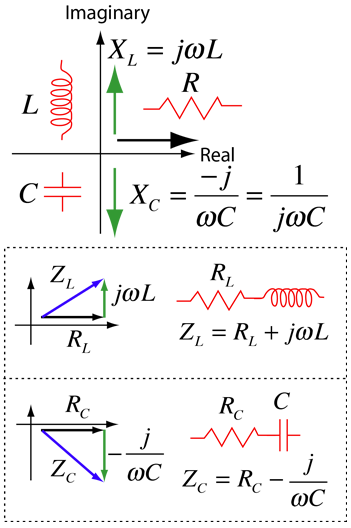# Use of Complex Impedance

The handling of the impedance of an AC circuit with multiple components quickly becomes unmanageable if sines and cosines are used to represent the voltages and currents. A mathematical construct which eases the difficulty is the use of complex exponential functions. The basic parts of the strategy are as follows:

 Math relationship underlying the techniqueEuler relation Polar form ofcomplex number The real part of a complex exponential function can be used to represent an AC voltage or current.The impedance can then be expressed as a complex exponential.Impedance combinations Phasor diagrams The impedance of the individual circuit elements can then be expressed as pure real or imaginary numbers.RL and RC combinations Example for parallel elements
Index

AC circuit concepts

 HyperPhysics***** Electricity and Magnetism R Nave
Go Back

# Complex Impedance for RL and RCUsing complex impedance is an important technique for handling multi-component AC circuits. If a complex plane is used with resistance along the real axis then the reactances of the capacitor and inductor are treated as imaginary numbers. For series combinations of components such as RL and RC combinations, the component values are added as if they were components of a vector. Shown here is the cartesian form of the complex impedance. They can also be written in polar form. Impedances in this form can be used as building blocks for calculating the impedances of combination circuits like the RLC parallel circuit.
 Impedance AC behavior of inductor AC behavior of capacitor
Index

AC circuit concepts

 HyperPhysics***** Electricity and Magnetism R Nave
Go Back

# Complex Impedance for RL and RC

This depicts the phasor diagrams and complex impedance expressions for RL and RC circuits in polar form. They can also be expressed in cartesian form.Impedance Polar form of complex number
Index

AC circuit concepts

 HyperPhysics***** Electricity and Magnetism R Nave
Go Back